# Complex numbers - math problems

#### Number of problems found: 28

• Evaluate 18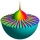Evaluate the expression (-4-7i)-(-6-9i) and write the result in the form a+bi (Real + i* Imaginary).
• Cis notation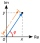Evaluate multiplication of two complex numbers in cis notation: (6 cis 120°)(4 cis 30°) Write result in cis and Re-Im notation.
• An Argand diagram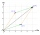Find the sum z1+z2 and difference z1-z2 and z2-z1 of z1=5+2i and z2=2+3i by an Argand diagram.
• Let 4Let z = 6 + 5i and w = 3 - i. Compute the following and express your answer in a + bi form. w + 3z
• Conjugate equation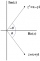Find the numbers of a and b, if (a - bi) (3 + 5i) is the Conjugate of (-6 - 24i)
• Eq2 equationsFor each of the following problems, determine the roots of the equation. Given the roots, sketch the graph and explain how your sketch matches the roots given and the form of the equation: g(x)=36x2-12x+5 h(x)=x2-4x+20 f(x)=4x2-24x+45 p(x)=9x2-36x+40
• Subtracting complex in polar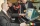Given w =√2(cosine (p/4) + i sine (pi/4) ) and z = 2 (cosine (pi/2) + i sine (pi/2) ), what is w - z expressed in polar form?
• Distance two imaginary numbsFind the distance between two complex number: z1=(-8+i) and z2=(-1+i).
• Modulus and argumentFind the mod z and argument z if z=i
• Linear combination of complexIf z1=5+3i and z2=4-2i, write the following in the form a+bi a) 4z1+6z2 b) z1*z2
• Two grandmothersTwo grandmothers went to sell eggs at the market, and they had a total of 100. When they sold all the eggs, they made the same money. The first grandmother says to the second: "If I sold my eggs for your price, I would earn 15 crowns. " The other grandmot
• Mappings of complex numbersFind the images of the following points under mappings: z=3-2j w=2zj+j-1
• Let z1=x1+y1i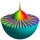Let z1=x1+y1i and z2=x2+y2i Find: a = Im (z1z2) b = Re (z1/z2)
• The modulus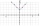Find the modulus of the complex number 2 + 5i
• Turtles 2A box turtle hibernates in the sand at 11 5/8. A spotted turtle hibernates at 11 16/25 feet. Which turtle is deeper? Write answer as number 1 or 2.
• Complex number coordinatesWhich coordinates show the location of -2+3i
• BearingA plane flew 50 km on a bearing 63°20' and the flew on a bearing 153°20' for 140km. Find the distance between the starting point and the ending point.
• Linear imaginary equationGiven that 2(z+i)=i(z+i) "this is z star" Find the value of the complex number z.A domed stadium is in the shape of spherical segment with a base radius of 150 m. The dome must contain a volume of 3500000 m³. Determine the height of the dome at its centre to the nearest tenth of a meter.
• Is complexAre these numbers 2i, 4i, 2i + 1, 8i, 2i + 3, 4 + 7i, 8i, 8i + 4, 5i, 6i, 3i complex?

Do you have an exciting math question or word problem that you can't solve? Ask a question or post a math problem, and we can try to solve it.

We will send a solution to your e-mail address. Solved examples are also published here. Please enter the e-mail correctly and check whether you don't have a full mailbox.

Please do not submit problems from current active competitions such as Mathematical Olympiad, correspondence seminars etc...

Try our complex numbers calculator.